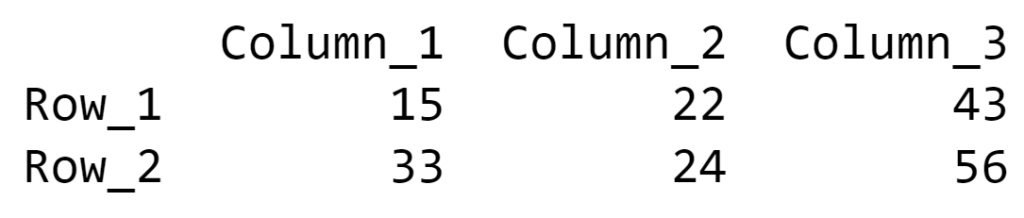Create a Pandas DataFrame from a Numpy array and specify the index column and column headers

• Difficulty Level : Medium
• Last Updated : 21 Aug, 2020

This article demonstrates multiple examples to convert the Numpy arrays into Pandas Dataframe and to specify the index column and column headers for the data frame.

Example 1: In this example, the Pandas dataframe will be generated and proper names of index column and column headers are mentioned in the function. This approach can be used when there is no pattern in naming the index column or column headers.

Below is the implementation:

Python3

 # Python program to Create a # Pandas DataFrame from a Numpy  # array and specify the index # column and column headers  # import required librariesimport numpy as npimport pandas as pd  # creating a numpy arraynumpyArray = np.array([[15, 22, 43],                        [33, 24, 56]])  # generating the Pandas dataframe# from the Numpy array and specifying# name of index and columnspanda_df = pd.DataFrame(data = numpyArray,                         index = ["Row_1", "Row_2"],                         columns = ["Column_1",                                   "Column_2", "Column_3"])  # printing the dataframeprint(panda_df)

Output:Example 2: In this example, the index column and column headers are generated through iteration. The range of iterations for rows and columns are defined by the shape of the Numpy array. With every iteration, a digit will be added to the predefined string and the new index column or column header will generate. Thus, if there is some pattern in naming the labels of the dataframe this approach is suitable.

Below is the implementation:

Python3

 # Python program to Create a # Pandas DataFrame from a Numpy  # array and specify the index column # and column headers  # import required librariesimport pandas as pdimport numpy as np  # creating a numpy arraynumpyArray = np.array([[15, 22, 43],                        [33, 24, 56]])  # generating the Pandas dataframe# from the Numpy array and specifying# name of index and columnspanda_df = pd.DataFrame(data = numpyArray[0:, 0:],                        index = ['Row_' + str(i + 1)                         for i in range(numpyArray.shape)],                        columns = ['Column_' + str(i + 1)                         for i in range(numpyArray.shape)])  # printing the dataframeprint(panda_df)

Output:Example 3: In this example, the index column and column headers are defined before converting the Numpy array into Pandas dataframe. The label names are again generated through iterations but the method is little different. Here, the number of iterations is defined by the length of the sub-array inside the Numpy array. This method can be used if the index column and column header names follow some pattern.

Below is the implementation:

Python3

 # Python program to Create a # Pandas DataFrame from a Numpy  # array and specify the index column # and column headers  # import required librariesimport pandas as pdimport numpy as np  # creating a numpy arraynumpyArray = np.array([[15, 22, 43],                        [33, 24, 56]])  # defining index for the # Pandas dataframeindex = ['Row_' + str(i)         for i in range(1, len(numpyArray) + 1)]  # defining column headers for the # Pandas dataframecolumns = ['Column_' + str(i)           for i in range(1, len(numpyArray) + 1)]  # generating the Pandas dataframe# from the Numpy array and specifying# details of index and column headerspanda_df = pd.DataFrame(numpyArray ,                         index = index,                        columns = columns)  # printing the dataframeprint(panda_df)

Output:Example #4: In this approach, the index column and the column headers for the Pandas dataframe will present itself in the Numpy array. During the conversion of the Numpy array into Pandas data frame, proper indexing for the sub-arrays of the Numpy array has to be done in order to get correct sequence of the dataframe labels.

Below is the implementation:

Python3

 # Python program to Create a # Pandas DataFrame from a Numpy  # array and specify the index column # and column headers  # import required librariesimport pandas as pdimport numpy as np  # creating a numpy array and# specifying the index and # column headers along with # data stored in the arraynumpyArray = np.array([['', 'Column_1',                        'Column_2', 'Column_3'],                       ['Row_1', 15, 22, 43],                       ['Row_2', 33, 24, 56]])  # generating the Pandas dataframe# from the Numpy array and specifying# details of index and column headerspanda_df = pd.DataFrame(data = numpyArray[1:, 1:],                        index = numpyArray[1:, 0],                        columns = numpyArray[0, 1:])  # printing the dataframeprint(panda_df)

Output:My Personal Notes arrow_drop_up